Question

# 14. Describe how you can test the hypothesis of Hardy-Weinberg equilibrium if all you know is...

14. Describe how you can test the hypothesis of Hardy-Weinberg equilibrium if all you know is observed genotype frequencies. Use complete sentences.

Let A be the dominant allele and a be recessive allele.

Observed Genotypes : AA, Aa, aa

p=freq A = ((observed AA count × 2) + observed Aa count) / (total genotypes count × 2)
q=freq a = ((observed aa count × 2) + observed Aa count) / (total genotypes count × 2)

The equation for genotype frequencies are

pAA = freq A² = p2
pAa = 2 × freq A × freq a = 2pq
paa = freq a² = q2

The expected frequencies are calculated as:

expAA = pAA × total genotypes count
expAa = pAa × total genotypes count
expaa = paa × total genotypes count

Using the above formula,  The Hardy-Weinberg equation is checked as:

p2 + 2pq + q2 = 1; p+q=1

Build a contingency table and calculate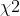table using the formula

X² = (obsAA-expAA)²/(expAA) + (obsaa-expaa)²/(expaa) + (obsAa-expAa)²/(expAa)

From this X² value, a p-value can be calculated for a X² distribution with 1 degree of freedom.

#### Earn Coins

Coins can be redeemed for fabulous gifts.

Similar Homework Help Questions
• ### 2. Hardy-Weinberg Equilibrium; chi-square test Sickle cell anemia is a recessive disorder caused by a recessive...

2. Hardy-Weinberg Equilibrium; chi-square test Sickle cell anemia is a recessive disorder caused by a recessive mutation (S) in the b-hemoglobin gene. 80% of affected SS individuals die before reproducing.   Heterozygotes (AS) and homozygous dominant (AA) individuals do not have sickle cell anemia. The table below shows the number of people of each genotype in a population of 100 people in population of Cameroon. Observed # individuals in a Cameroon population AA AS SS 62 32 6 What are the...

• ### EXERCISE 6 HARDY-WEINBERG EQUILIBRIUM Work in a small group or alone to complete this exercise. In...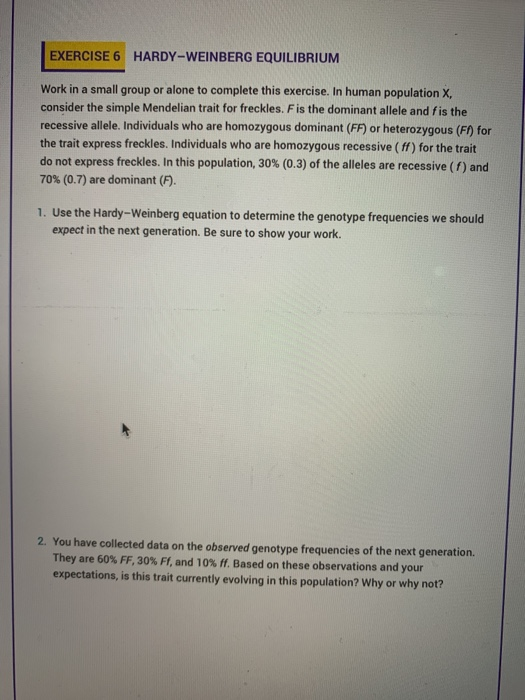EXERCISE 6 HARDY-WEINBERG EQUILIBRIUM Work in a small group or alone to complete this exercise. In human population X consider the simple Mendelian trait for freckles. F is the dominant allele and fis the recessive allele. Individuals who are homozygous dominant (FF) or heterozygous (F) for the trait express freckles. Individuals who are homozygous recessive (ff) for the trait do not express freckles. In this population, 30% (0.3) of the alleles are recessive (1) and 70% (0.7) are dominant (F)....

• ### In a population of wild sweet pea plants that you assume are in Hardy-Weinberg equilibrium, 1%...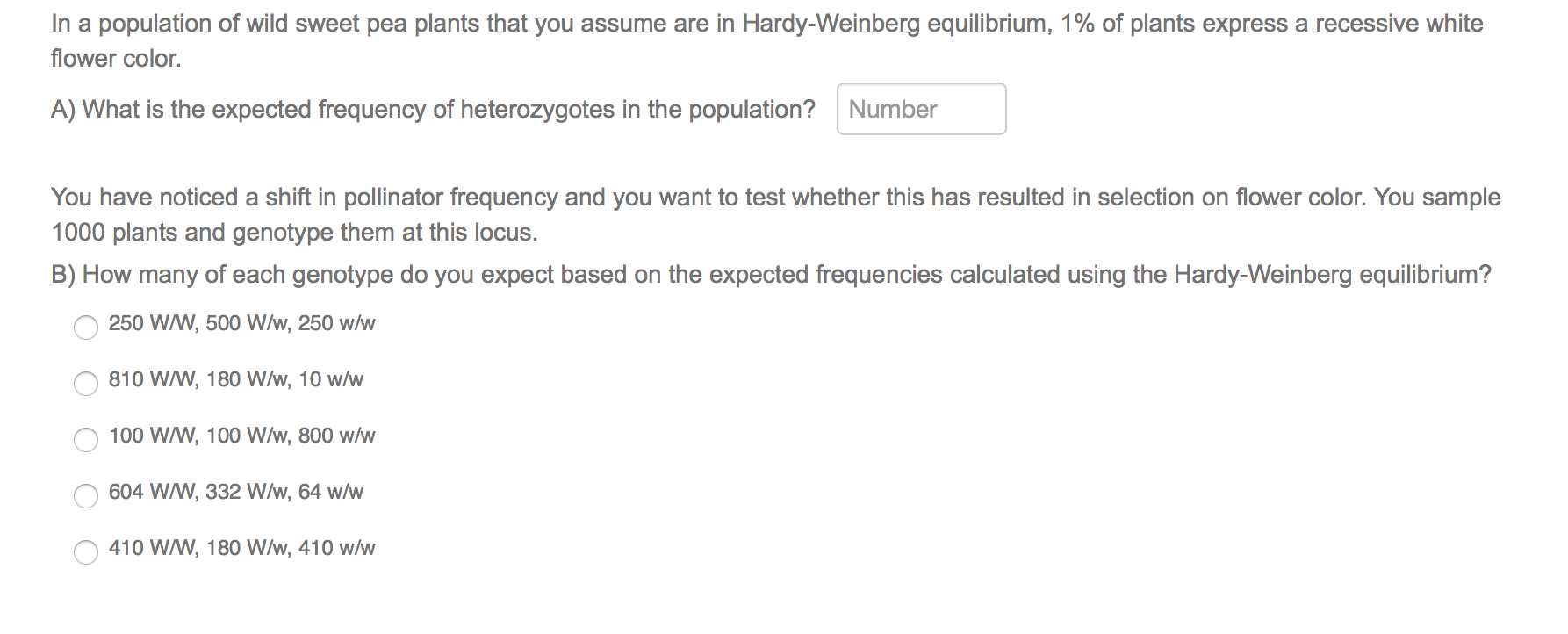In a population of wild sweet pea plants that you assume are in Hardy-Weinberg equilibrium, 1% of plants express a recessive white flower color. A) What is the expected frequency of heterozygotes in the population? Number You have noticed a shift in pollinator frequency and you want to test whether this has resulted in selection on flower color. You sample 1000 plants and genotype them at this locus. B) How many of each genotype do you expect based on the...

• ### Hardy-Weinberg a. if you know allelic frequencies for a particular gene in your class, can you...

Hardy-Weinberg a. if you know allelic frequencies for a particular gene in your class, can you calculate phenotypic frequencies. why or why not b. if you know allelic frequencies for a population, can you always calculate phenotypic frequencies. c. if the fraction of homozygous recessive in a population is known, can the allelic frequencies and genotypic frequencies always be calculated? what conditions must be met?

• ### reting Data: Hardy-Weinberg Equation 2 of 10 you use the Hardy Weinberg equation to answer questions...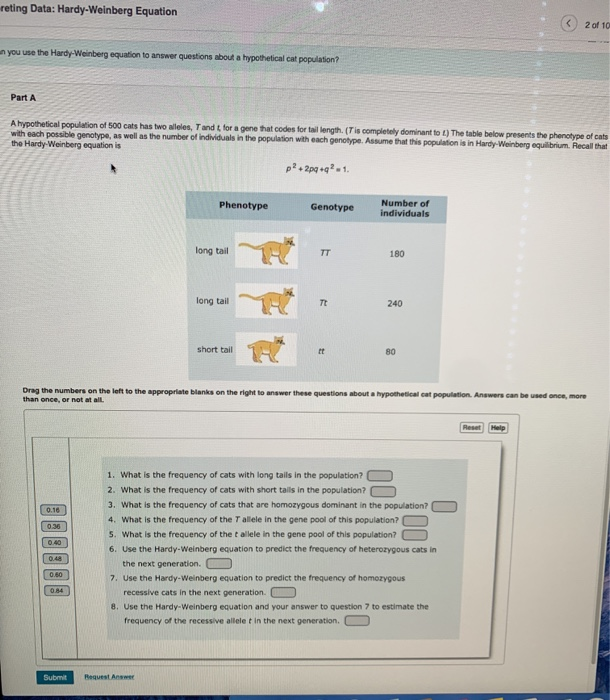reting Data: Hardy-Weinberg Equation 2 of 10 you use the Hardy Weinberg equation to answer questions about a hypotheticalcat population Part A A hypothetical population of 500 cats has two wees, Tandt for a gene that codes for tail length (Tis completely dominantot) The table below presents the phenotype of cats with each possible genotype, as well as the number of individuals in the population with each genotype. Assume that this population is in Hardy-Weinberg equilibrium Recall that the Hardy...

• ### a. Which of the following two large populations is in Hardy-Weinberg Equilibrium with respect to the...

a. Which of the following two large populations is in Hardy-Weinberg Equilibrium with respect to the A locus? Genotype frequencies AA Aa aa Population I .430 .481 .089 Population II .640 .320 .040 b. What are the expected equilibrium frequencies for the population that is not in equilibrium? c. How long will it take for the non-equilibrium population to reach equilibrium under conditions of random mating and assuming no selection at this locus?

• ### please click on the photo to see all of it The basic equations of Hardy-Weinberg Equilibrium...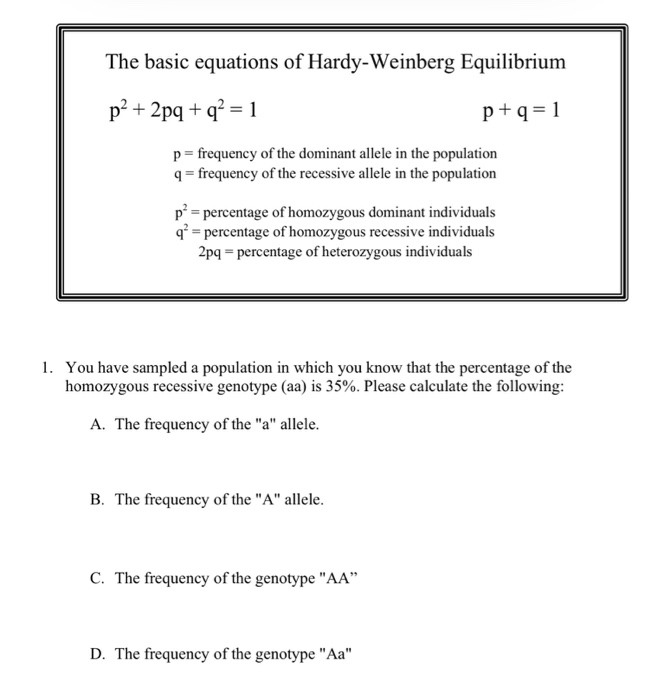please click on the photo to see all of it The basic equations of Hardy-Weinberg Equilibrium p² + 2pq + q2 = 1 p+q=1 p= frequency of the dominant allele in the population 9 = frequency of the recessive allele in the population př= percentage of homozygous dominant individuals q* = percentage of homozygous recessive individuals 2pq - percentage of heterozygous individuals 1. You have sampled a population in which you know that the percentage of the homozygous recessive genotype...

• ### Consider a population with two autosomal alleles A, a, in Hardy-Weinberg equilibrium Let p and q...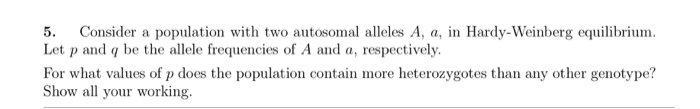Consider a population with two autosomal alleles A, a, in Hardy-Weinberg equilibrium Let p and q be the allele frequencies of A and a, respectively 5. For what values ofp does the population contain more heterozygotes than any other genotype? Show all your working

• ### Population Genetics and Human Evolution Complete the following discussions: The Hardy-Weinberg equilibrium states that allele frequencies...

Population Genetics and Human Evolution Complete the following discussions: The Hardy-Weinberg equilibrium states that allele frequencies remain constant across generations unless certain influences are introduced, such as nonrandom matings or mutations. Describe the Hardy-Weinberg principle. Are there influences that deviate from the principle? If so, what are they? If no, why?

• ### 2.3 Problem 3 The Hardy-Weinberg equation is useful for predicting the percent of a hu- man...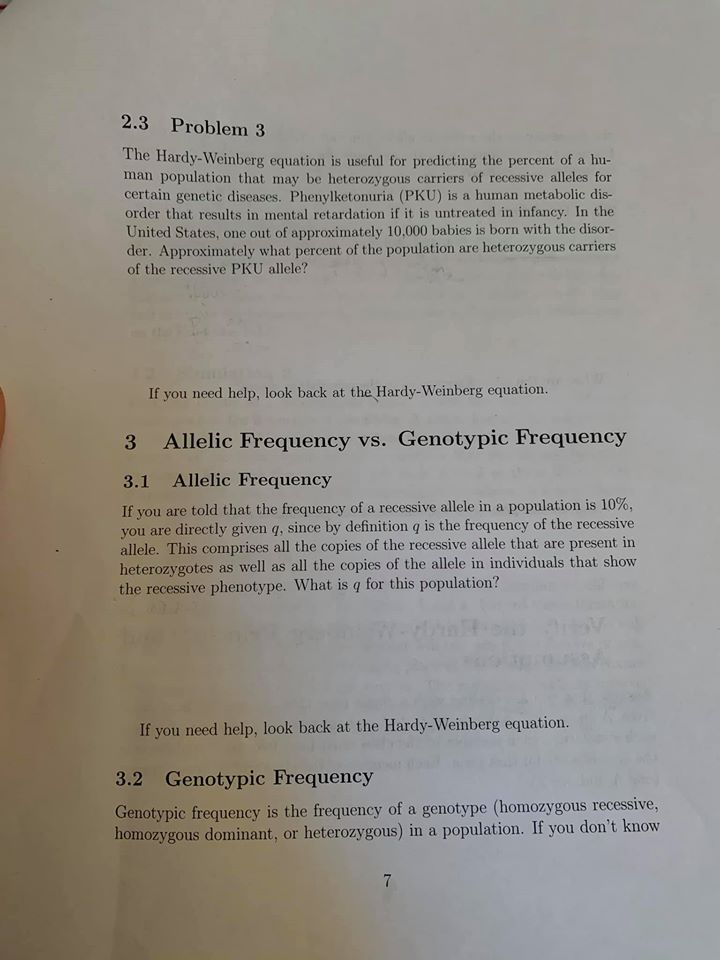2.3 Problem 3 The Hardy-Weinberg equation is useful for predicting the percent of a hu- man population that may be heterozygous carriers of recessive alleles for certain genetic diseases. Phenylketonuria (PKU) is a human metabolic dis- order that results in mental retardation if it is untreated in infancy. In the United States, one out of approximately 10.000 babies is born with the disor- der. Approximately what percent of the population are heterozygous carriers of the recessive PKU allele? If you...